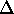# Lenses Prelab Assignment

Name:_______________________________ 4-digit Code Number:_______________________________

1. Describe a quick way to tell a converging lens from a diverging lens with no equipment.
2. Derive the formula for error in the focal length: (f) / f2    =    (do) / do2    +    (di) / di2 from the lens grinder's equation.
3. Describe in detail the two methods for determining the focal length of a diverging lens which are used in this lab.
4. What is a third method for determining the focal length of a diverging lens which is not used in this lab?

Go to the lab